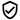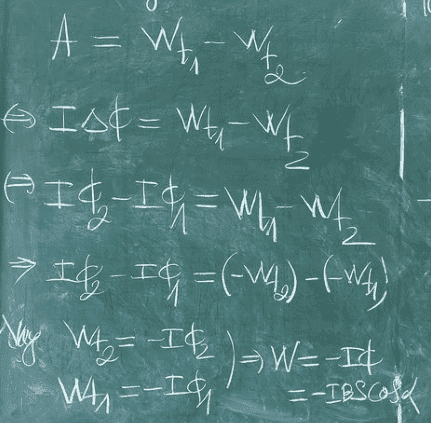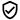# Why is the energy of a circuit placed in a magnetic field at infinity equal to zero?

qh4305
Why is the energy of a circuit placed in a magnetic field at infinity zero?Mentor
Because the batteries get drained on the way there? It's a long trip, after all.But seriously, what are you talking about? Are you confusing gravitational potential energy (GPE) with electrical energy?

qh4305
Because the batteries get drained on the way there? It's a long trip, after all.But seriously, what are you talking about? Are you confusing gravitational potential energy (GPE) with electrical energy?
I had a bit of a problem with proving a formula, and I made myself an assumption that the energy of the circuit placed in a magnetic field at infinity would be zero, but maybe that doesn't hold true for science, engineering, and so on. The formula that I am proving is the formula for calculating the magnetic energy of a closed circuit in a magnetic field, I used the convention that the work of the magnetic force is equal to the decrease in potential energy, but now I think it is not quite right. Can you help me prove this energy formula?
p/s:
sorry for my englishGold Member
I had a bit of a problem with proving a formula,

Gold Member
I think we are all confused about the scenario you are asking about. What's at infinity, compared to what? What sort of magnetic field? What made it? What do you mean by a circuit? Is it just a loop of wire?

Maybe a simple sketch would help.

•qh4305 and davenn
qh4305thanks.

qh4305Is this a correct proof?